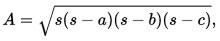# How to compute the area of any type of land practically at the job siteIn this construction video tutorial, one can learn how to work out v-shaped land area practically at the construction site.

The calculation process is based on Heron’s Formula. This formula is also known as Hero’s formula.

This formula can be applied to detect the area of a triangle with the 3 side lengths.

So, it is not necessary to depend on the formula for area that employs base and height.

Heron's formula defines the area of a triangle whose sides contain lengths a, b, and c and represented as follow :-

AREA =Here, s represents the semiperimeter of the triangle;
S = a+b+c/2

By applying this formula, you can calculate any type of area except curve shaped area.

To get the detailed process, watch the following video.